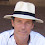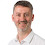## Tuesday, 18 August 2009

There is a very simple explanation for using the "=" sign in the expression builder - it tells QlikView to evaluate everything after the "=" and return the result.

Where there is some confusion is that within charts (and, in successive versions it has been removed in other places) the "=" is not necessary because it is assumed - you wouldn't put an expression in a chart unless you wanted it to be evaluated, would you?

In a text box, the need for the "=" becomes more apparent. If I add a Text Box to my layout and set the text expression to:

Sum(Sales)

Then the Text Box will come to the screen and have a value of "Sum(Sales)". This makes sense because the default interpretation of a Text Box should be that the values in the expression are just text. Now, if I add the "=" into the expression:

=Sum(Sales)

Then the calculation is performed and the result, the sum of all sales based on current selections in the document, is displayed in the Text Box.

It becomes more interesting when we use, or do not use, the "=" in a variable.

For example, if I set up 2 variables, vSales and vTotalSales.

vSales is going to have a value set up in the Expression Builder of:

Sum(Sales)

vTotalSales is going to have a value set up in the Builder of:

=Sum(Sales)

Now, if I set up a straight table and add a dimension, say "Year", and then add 2 expressions - one that is just \$(vSales) and the other is \$(vTotalSales), then the first column will show sales by Year and the second will show the same value in each row - the total sales for the document.

This happens because the first variable is simply a piece of text - "Sum(Sales)" - that is replaced into the chart expression and evaluated against each dimension. The "=" in the second variable causes it to be evaluated at the document level and it is the result rather than the expression that is inserted into the chart.

The "=" is also interesting in Dollar Expansion. We could achieve the same result as vTotalSales in the chart by using the expression:

\$(=Sum(Sales))

This Sum(Sales) is now calculated outside the chart - i.e. at the document level - and the result, not the expression, is returned to the chart.

Knowing about the "=" sign in Expression Builder is very useful to avoid confusion and also to give you a new weapon in your arsenal.

1.Great post Stephen. Your examples are very useful.

-Rob

2.Thanks Rob.

Anyone who doesn't know of Rob should go and check out his excellent QlikView site and download the Cookbook: